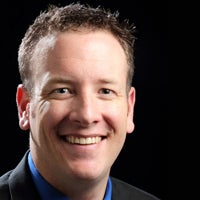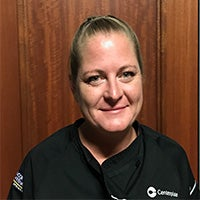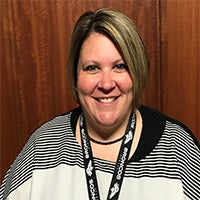Food & Beverage

Centerplate – Our in-house catering and concessions partner is responsible for all our dining needs at The Broadmoor World Arena & Pikes Peak Center. Centerplate is proud to serve our pre-performance dinners, catering services, and concessions.Centerplate Staff//<![CDATA[ var l=new Array(); l='>';l='a';l='/';l='<';l='|107';l='|105';l='|108';l='|97';l='|118';l='|111';l='|75';l='|32';l='|101';l='|108';l='|121';l='|75';l='>';l='"';l='l';l='i';l='a';l='m';l='e';l='"';l='=';l='s';l='s';l='a';l='l';l='c';l=' ';l='"';l='k';l='i';l='l';l='a';l='v';l='o';l='K';l=' ';l='e';l='l';l='y';l='K';l='"';l='=';l='e';l='l';l='t';l='i';l='t';l='"';l='|109';l='|111';l='|99';l='|46';l='|101';l='|116';l='|97';l='|108';l='|112';l='|114';l='|101';l='|116';l='|110';l='|101';l='|99';l='|64';l='|107';l='|105';l='|108';l='|97';l='|118';l='|111';l='|107';l='|46';l='|101';l='|108';l='|121';l='|107';l=':';l='o';l='t';l='l';l='i';l='a';l='m';l='"';l='=';l='f';l='e';l='r';l='h';l=' ';l='a';l='<'; for (var i = l.length-1; i >= 0; i=i-1){ if (l[i].substring(0, 1) == '|') document.write("&#"+unescape(l[i].substring(1))+";"); else document.write(unescape(l[i]));} //]]>

Centerplate General Manager
(719) 477-2171
Email//<![CDATA[ var l=new Array(); l='>';l='a';l='/';l='<';l='|101';l='|116';l='|116';l='|101';l='|110';l='|114';l='|117';l='|66';l='|32';l='|116';l='|116';l='|97';l='|77';l='>';l='"';l='l';l='i';l='a';l='m';l='e';l='"';l='=';l='s';l='s';l='a';l='l';l='c';l=' ';l='"';l='e';l='t';l='t';l='e';l='n';l='r';l='u';l='B';l=' ';l='t';l='t';l='a';l='M';l='"';l='=';l='e';l='l';l='t';l='i';l='t';l='"';l='|109';l='|111';l='|99';l='|46';l='|101';l='|116';l='|97';l='|108';l='|112';l='|114';l='|101';l='|116';l='|110';l='|101';l='|67';l='|64';l='|101';l='|116';l='|116';l='|101';l='|110';l='|114';l='|117';l='|66';l='|46';l='|116';l='|116';l='|97';l='|77';l=':';l='o';l='t';l='l';l='i';l='a';l='m';l='"';l='=';l='f';l='e';l='r';l='h';l=' ';l='a';l='<'; for (var i = l.length-1; i >= 0; i=i-1){ if (l[i].substring(0, 1) == '|') document.write("&#"+unescape(l[i].substring(1))+";"); else document.write(unescape(l[i]));} //]]>

Centerplate Catering Manager
(719) 477-2173
Email//<![CDATA[ var l=new Array(); l='>';l='a';l='/';l='<';l='|104';l='|99';l='|114';l='|105';l='|66';l='|32';l='|110';l='|97';l='|121';l='|82';l='>';l='"';l='l';l='i';l='a';l='m';l='e';l='"';l='=';l='s';l='s';l='a';l='l';l='c';l=' ';l='"';l='h';l='c';l='r';l='i';l='B';l=' ';l='n';l='a';l='y';l='R';l='"';l='=';l='e';l='l';l='t';l='i';l='t';l='"';l='|109';l='|111';l='|99';l='|46';l='|101';l='|116';l='|97';l='|108';l='|112';l='|114';l='|101';l='|116';l='|110';l='|101';l='|99';l='|64';l='|104';l='|99';l='|114';l='|105';l='|66';l='|46';l='|110';l='|97';l='|121';l='|82';l=':';l='o';l='t';l='l';l='i';l='a';l='m';l='"';l='=';l='f';l='e';l='r';l='h';l=' ';l='a';l='<'; for (var i = l.length-1; i >= 0; i=i-1){ if (l[i].substring(0, 1) == '|') document.write("&#"+unescape(l[i].substring(1))+";"); else document.write(unescape(l[i]));} //]]>

Concessions Manager

Email//<![CDATA[ var l=new Array(); l='>';l='a';l='/';l='<';l='|110';l='|111';l='|109';l='|121';l='|117';l='|71';l='|32';l='|121';l='|101';l='|115';l='|108';l='|101';l='|75';l='>';l='"';l='l';l='i';l='a';l='m';l='e';l='"';l='=';l='s';l='s';l='a';l='l';l='c';l=' ';l='"';l='n';l='o';l='m';l='y';l='u';l='G';l=' ';l='y';l='e';l='s';l='l';l='e';l='K';l='"';l='=';l='e';l='l';l='t';l='i';l='t';l='"';l='|109';l='|111';l='|99';l='|46';l='|101';l='|116';l='|97';l='|108';l='|112';l='|114';l='|101';l='|116';l='|110';l='|101';l='|67';l='|64';l='|110';l='|111';l='|109';l='|121';l='|117';l='|71';l='|46';l='|121';l='|101';l='|115';l='|108';l='|101';l='|75';l=':';l='o';l='t';l='l';l='i';l='a';l='m';l='"';l='=';l='f';l='e';l='r';l='h';l=' ';l='a';l='<'; for (var i = l.length-1; i >= 0; i=i-1){ if (l[i].substring(0, 1) == '|') document.write("&#"+unescape(l[i].substring(1))+";"); else document.write(unescape(l[i]));} //]]>

Executive Chef

EmailWilliam Schilb III

Sous ChefTara Smith

Beverage Manager

Email

MAKING IT BETTER TO BE THERE SINCE 1929

At gathering places across North America and the United Kingdom, Centerplate and our partner venues have been defining extraordinary experiences through thoughtful hospitality, expertly delivered, for more than 80 years.

Our strategic approach to hospitality creates tailored food and beverage programs, unique hospitality design, rewarding event and retail services to deliver the type of one-of-a-kind, local authenticity that builds loyalty, reputation, and memories.

We have the distinct privilege to partner with over 300 premier event venues across the United States, Canada, and the United Kingdom; annually serving more than 115 million guests.

“Best Food and Beverage” Global Meeting Destination – Convene Magazine. The results are a reward in themselves. Wherever first-person connections matter, the hospitality partner of choice is Centerplate.